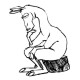4:13 AM
0I want to show that for an anti-symmetric two upper index tensor $S^{ab}$, that: $$Q^{bcd}=S^{a[b} \nabla_a S^{cd]}=0$$ I'm trying to figure out how to show prove the hint equality is true using the penrose diagrammatic notation. I've tried to make a diagram of it below: Could someone explain me...

21Penrose graphical notation seems to be a convenient way to do calculations involving tensors/ multilinear functions. However the wiki page does not actually tell us how to use the notation. The several references, especially ones with Penrose as author, must be good places to start. But it is no...

20For two matrices $\textbf{S}$ and $\textbf{T}$, a proof of $\det(\textbf{ST})=\det(\textbf{S})\det(\textbf{T})$ is given below in the diagrammatic tensor notation. Here $\det$ denotes the determinant. Why can the antisymmetrizing bar be inserted in the middle because "there is already antisym...Penrose graphical notation
In mathematics and physics, Penrose graphical notation or tensor diagram notation is a (usually handwritten) visual depiction of multilinear functions or tensors proposed by Roger Penrose in 1971. A diagram in the notation consists of several shapes linked together by lines. The notation has been studied extensively by Predrag Cvitanović, who used it, Feynman's diagrams and other related notations in developing birdtracks (a group-theoretical version of Feynman diagrams) to classify the classical Lie groups. Penrose's notation has also been generalized using representation theory to spin networks...

16 hours later…8:06 PM
A new tag was created. There is a tag-excerpt, too.
0I have been reading commutative algebra from lecture notes and I have some questions in a proof of a corollary of Hilbert Nullstellansatz. Let R be a finitely generated k-algebra. Then for an ideal $I \subseteq R$, $\sqrt{I} =\cap M$, where M are maximal ideals containing I. Proof: Clearly $\s...Hilbert's Nullstellensatz In mathematics, Hilbert's Nullstellensatz (German for "theorem of zeros," or more literally, "zero-locus-theorem"—see Satz) is a theorem that establishes a fundamental relationship between geometry and algebra. This relationship is the basis of algebraic geometry. It relates algebraic sets to ideals in polynomial rings over algebraically closed fields. This relationship was discovered by David Hilbert, who proved the Nullstellensatz and several other important related theorems named after him (like Hilbert's basis theorem). == Formulation == Let k be a field (such as the rational numbers) and K... 4Consider a continuous time dynamical system where $$\dot x(t) = F(x(t))$$, where$x(t)$is a coordinate vector of state and the right side of the equation$F$is a non-linear smooth function. Let the state space be Euclidean. Let$S^t(x_0)$be the position of the trajectory of$\$\dot x(t)= F(x(t)...9:01 PM
The tag seems to have been created recently, but not picked up above. Not sure what the purpose is, and would support deleting it.